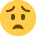# actual usage of materials in the variance analysis

Hi there

I have been given the following information
materials std unit of input is 10 litres,
standard price per unit of imput is £2,
std cost per unit is £20,
std budgeted units of production (5000 units of 10 litres drums).
std cost of budgeted production £100,000

this says to me that there are 100 litres that go into a standard unit of production (a std unit being a 10 litres drum) therefore I see that 90 litres is wasted in production or something

Actual then is
£2.05 per unit of input (ie per 10 litres)
actual cost is £105,000

and what was actually produced was 4800 units

The question asks what was the actual usage of materials in litres.
Since it costs £2.05 for 10 litres then it follows it costs .205 p for 1 litres
so I divided £105,000 by /.205p to try to get the litres.

this comes out at an odd number
ie 512,195.12 litres

Am I calculating this correctly.
we are not told the actual amount of materials I need to calculate it.

If we calculate it should we be using the actual units produced to work out the actual usage and the costs are actually irrelevant.

as part of material usage variance calculation we need to
calculate the actual quantity used @ std price.

My question therefore is
is the actual quantity used in this calculation - the actual quantity used in production inlcuding any spilages or spoilt materials or just the actual stuff that went into the production (which we actually dont know)

many thanks

Karen

• From this isnt it only 10 litres that goes into 1 unit. Where are you getting 100 litres from?
• Hello,

Here's my attempt, not done standard costing for a while so someone else may need to verify my answer:

Standard Cost for 1 Unit = 10 litres @ £2 = £20

Total Material Cost Variance for actual production:
Standard: 4,800 Units @ £ 20 = 96,000
Actual:..............................= 105,000
Variance:............................= 9,000 (A)

With information we have we can work out the Material Price Variance:
Standard: 4,800 Units * 10 litres = 48,000 litres @ £2 = 96,000
Actual:......................................48,000 litres @ £ 2.05 = 98,400
Variance:...............................................................= 2,400 (A)

From above you can see that out of a total variance of 9,000 (A), 2,400 (A) was caused by Material price so therefore 6,600 (A) (9,000 - 2,400) must be caused my Material usage variance.

Material Usage Variance:
Standard 4,800 Units * 10 litres = 48,000 litres @ £ 2 = 96,000
Total: £ 102,600

Materials actually used = £ 102,600 / £ 2 = 51,300 litres
• Apologies, pls ignore above I think it is actually just as simple as £ 105,000 / £ 2.05 = 51,220 litres used.

Material Price Variance 2,560 (A) (51,220 * £2) - (51,220 * £2.05)
Material Usage Variance 6,440 (A) (48,000 * £2) - (51,220 * £2)
Total Material Variance 9,000 (A)

Sorry for any confusion• Hi Guys

yes I did 105000/.205 = 512195.12 litres
its £2.05 for 10 litres and they want to know how many litres were actually used.

I dont have the actual number only that 4800 were produced. usually we are given the actual litres used. So this is all I have. Its just that it works out so odd everything else in the question works out well

anyway guys thanks for that will go with this

Karen